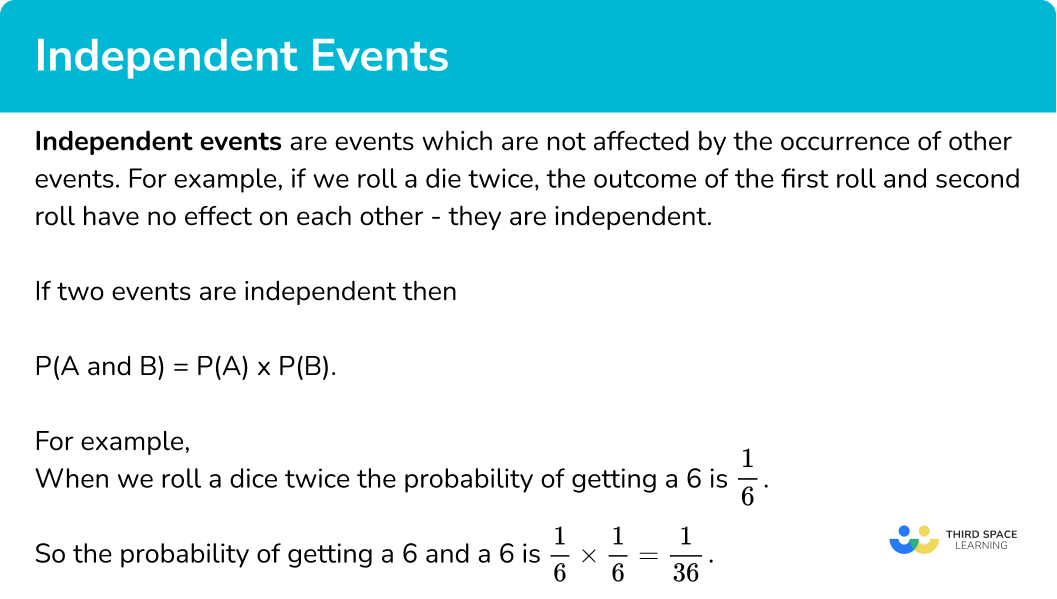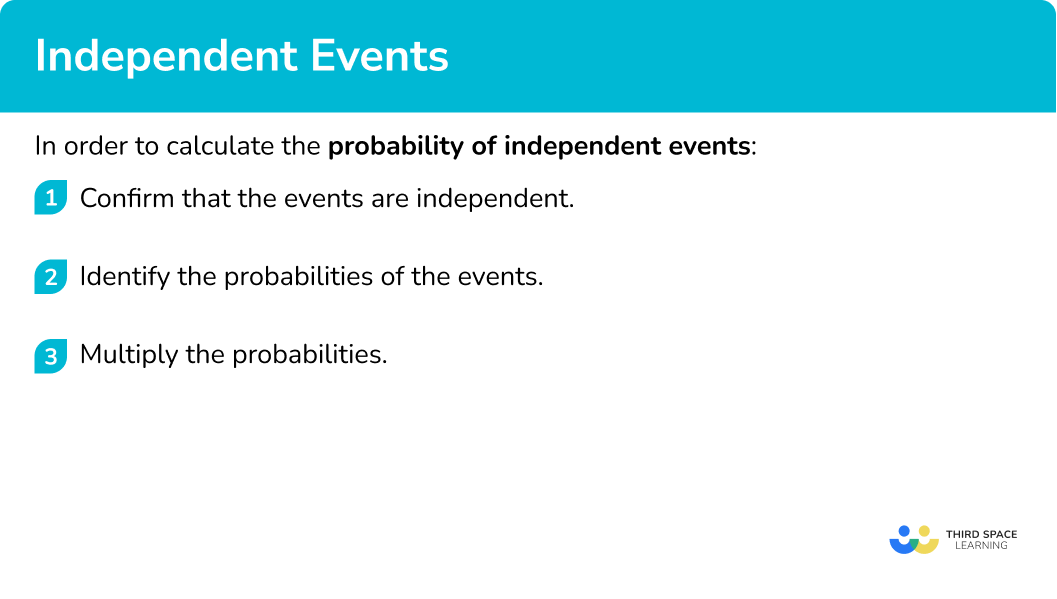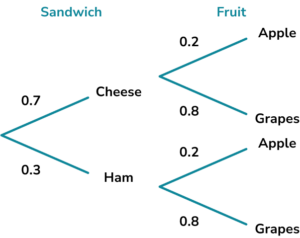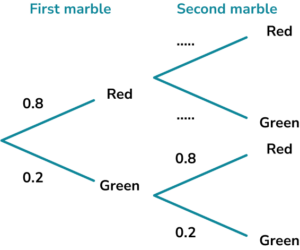# Independent Events

Here we will learn about independent events, including what independent events are and how to calculate the probability of independent events occurring.

There are also calculating probability worksheets based on Edexcel, AQA and OCR exam questions, along with further guidance on where to go next if you’re still stuck.

## What are independent events?

Independent events are events which are not affected by the occurrence of other events. For example, if we roll a die twice, the outcome of the first roll and second roll have no effect on each other – they are independent.

If two events are independent then

\textbf{P(A} and \textbf{B) = P(A) × P(B)} .

This is called the multiplication rule.

Let’s look at an example.

If we are rolling a dice twice, we can find the probability of getting two sixes.

The probability of getting a 6 is \frac{1}{6} .

So the probability of getting a 6 and a 6 is

\frac{1}{6} \times\frac{1}{6} = \frac{1}{36}.

Step-by-step guide: Combined events probability

### What are independent events?## How to calculate the probability of independent events

In order to calculate the probability of independent events:

1. Confirm that the events are independent.
2. Identify the probabilities of the events.
3. Multiply the probabilities.

### Explain how to calculate the probability of independent events## Independent event examples

### Example 1: identifying independent events

Look at the events below. Which set of events are not independent?

• Rolling a die and getting a 6 and tossing a coin and getting a head.
• Tossing two coins and getting two heads.
• Choosing a blue ball from a bag containing blue and yellow balls, not replacing it, and then choosing another blue ball from the same bag.
• A student in class 5 having brown hair and size 3 feet.

The definition of independence is that the events have no effect on each other.

When you roll a die and toss a coin, the outcome of one does not affect the outcome of the other therefore the events are independent

When you toss two coins, the outcome of one does not affect the other therefore the events are independent.

When you choose a ball from a bag and do not replace it, you are changing the number of balls in the bag and therefore the outcome of choosing the first ball will have an effect on the outcome of choosing the second ball (for example, if you choose a blue ball the first time, the probability of choosing a blue ball the second time will decrease). Therefore these are dependent events.

The colour of someone’s hair does not affect the size of their feet therefore the events are independent.

### Example 2: probability of two independent events

The probability that Tom forgets his PE kit is 0.3. The probability that Noah forgets his PE kit is 0.1. The events are independent.

Calculate the probability that both Tom and Noah forget their PE kits on the same day.

We are told in the question that the events are independent.

The first event is that Tom forgets his PE kit. The probability that Tom forgets his PE kit is 0.3.

The second event is that Noah forgets his PE kit. The probability that Noah forgets his PE kit is 0.1.

The probability that Tom and Noah both forget their PE kits is

0.3 \times 0.1 = 0.03.

### Example 3: probability of three independent events

Rachel tosses three fair coins. Find the probability that all three coins land on tails.

The outcome for each coin is not affected by the other coins therefore the events are independent.

Since the coins are fair, for each coin the probability that it lands on tails is \frac{1}{2}.

The probability of getting three tails is

\frac{1}{2} \times \frac{1}{2} \times \frac{1}{2} = \frac{1}{8}.

### Example 4: using a tree diagram

Niamh plays a game of table tennis on Saturday and a game of badminton on Sunday. The different outcomes are represented on the tree diagram below.

Calculate the probability that Niamh wins both games.

The outcome of one game does not affect the outcome of the other game therefore the events are independent.

The probability that Niamh wins table tennis is 0.4 and the probability that Niamh wins badminton is 0.7.

The probability that Niamh wins table tennis and badminton is

0.4 \times 0.7=0.28.

### Example 5: finding the probability of individual events

Zainab has two bags of mixed sweets. She picks one sweet from each bag. The probability that Zainab picks a lollipop from the first bag is \frac{1}{6} and the probability that she picks a lollipop from both bags is \frac{1}{15} .

What is the probability that she picks a lollipop from the second bag?

The type of sweet that is picked from one bag has no effect on the sweet picked from the other bag therefore the events are independent.

The probability that Zainab picks a lollipop from the first bag is \frac{1}{6} and the probability that she picks a lollipop from both bags is \frac{1}{15}.

If we call the probability of picking a lollipop from the second bag \text{P(L)} then

\frac{1}{6} \times \text{P(L)}=\frac{1}{15}.

Solving this

\begin{aligned} \text{P(L)}&=\frac{1}{15} \div\frac{1}{6}\\\\ &=\frac{1}{15} \times \frac{6}{1}\\\\ &=\frac{6}{15} \text{ or } \frac{2}{5} \end{aligned}

### Example 6: finding the probability of individual events

Rosie flips a biased coin twice. The probability that Rosie gets two heads is 0.64.

Find the probability that Rosie gets a head on a single coin flip.

The results of the first coin flip will not affect the second coin flip so the events are independent.

Here we only know that the probability that Rosie gets two heads is 0.64.

If we call the probability that Rosie gets a head \text{P(H)} then

\text{P(H)} \times \text{P(H)} = 0.64.

Solving this

\begin{aligned} \text{(P(H))}^{2}&=0.64\\\\ \text{P(H)}&=\sqrt{0.64}\\\\ \text{P(H)}&=0.8 \end{aligned}

The probability of getting a head on a single coin flip is 0.8.

### Common misconceptions

• Multiplying or dividing fractions incorrectly

To multiply fraction, multiply the numerators and multiply the denominators. To divide fractions, turn the second one upside down and multiply.

For independent events P(A and B) = P(A) \times P(B).

For mutually exclusive events P(A or B) = P(A) + P(B).

### Practice independent events questions

1. Identify the pair of events that are not independent.

Rolling two dice and each landing on 6.Rain falling on 20th December 2020 and 20th December 2021.Flipping a coin and landing on tails and picking a king from a deck of cards.Picking one card from a deck of cards and then a second card and getting an ace both times.If you choose one card from a deck of cards, you are changing the number of cards left and therefore the probabilities of each outcome for the next draw.

Picking one card followed by a second card from a deck of cards are not independent events.

2. Events A and B are shown below.

Event A – rolling a die and landing on an even number.

Event B – picking a diamond from a deck of cards.

Felicity rolls a die and picks a card from a deck of cards. Find the probability of both events A and B occurring.

\frac{3}{4}\frac{1}{8}\frac{1}{2}\frac{1}{4}The probability of a die landing on an even number is \frac{1}{2}.

The probability of picking a diamond from a deck of cards is \frac{1}{4}.

The probability of events A and B both occurring is

\frac{1}{2} \times \frac{1}{4} = \frac{1}{8}.

3. The probability that my train is late on any given day is 0.3. Find the probability that my train is late three days in a row.

0.30.90.0270.27The probability that the train is late on any one day is 0.3.

The probability that the train is late on day 1 and day 2 and day 3 is

0.3 \times 0.3 \times 0.3 = 0.027.

4. Sam has different options for her lunch. The probabilities of her choosing the different options are shown below.Find the probability that Sam chooses a cheese sandwich and grapes.

0.561.50.150.06The probability that Sam chooses a cheese sandwich is 0.7 and the probability that Sam chooses grapes is 0.8.

The probability that Sam chooses a cheese sandwich and grapes is

0.7 \times 0.8=0.56.

5. Sheila and Pete are playing darts. The probability that Sheila hits a bullseye is 0.1 and the probability that they both hit a bullseye is 0.02. Find the probability that Pete hits a bullseye.

0.0020.20.120.3If we call the probability that Pete hits a bullseye \text{P(P)} then

\begin{aligned} 0.1 \times \text{P(P)}&=0.02\\\\ \text{P(P)}&=0.02 \div 0.1\\\\ &=0.2 \end{aligned}

6. Marwa rolls a biased die twice. The probability that she gets two sixes is \frac{4}{25}. Find the probability that Marwa rolls a six on any one roll.

\frac{2}{25}\frac{2}{5}\frac{8}{25}\frac{16}{125}If we call the probability of rolling a six P(6) then

\begin{aligned} \text{P(6)} \times \text{P(6)}&=\frac{4}{25}\\\\ \text{(P(6))}^{2}&=\frac{4}{25}\\\\ \text{P(6)}&=\sqrt{\frac{4}{25}}\\\\ \text{P(6)}&=\frac{2}{5} \end{aligned}

### Independent events GCSE questions

1. Eleri rolls a die and tosses two coins. Find the probability that Eleri gets a 4 on the die and heads on both coins.

(2 marks)

\frac{1}{6} \times \frac{1}{2} \times \frac{1}{2}

(1)

=\frac{1}{24}

(1)

2. (a) A marble is picked from a bag containing red and green marbles. The marble is replaced and a second marble is then picked. The tree diagram below shows the possible outcomes.Fill in the missing numbers on the tree diagram.

(b) Find the probability that two green marbles are picked.

(3 marks)

(a)

Red 0.8 and green 0.2

(1)

(b)

0.2 \times 0.2

(1)

0.04

(1)

3. Rosie rolls a biased die twice. The probability that she gets an even number on both rolls is 0.16.

Find the probability that she gets an odd number on both rolls.

(4 marks)

(P( even number ))^2=0.16

(1)

P( even number )=0.4

(1)

P( odd number )=0.6

(1)

P( odd number and odd number )=0.6^{2}=0.36

(1)

## Learning checklist

You have now learned how to:

• Identify independent events
• Calculate the probability of independent events

## Still stuck?

Prepare your KS4 students for maths GCSEs success with Third Space Learning. Weekly online one to one GCSE maths revision lessons delivered by expert maths tutors.

Find out more about our GCSE maths tuition programme.